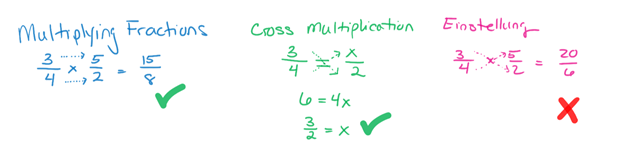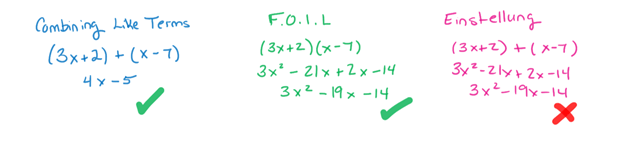# Effective Study Skills Part 4 – EinstellungIf you take practicing too far, you might end up experiencing the dreaded Einstellung Effect. This means that you learn one process so well that you use it even when you’re not supposed to. Einstellung is the German word for “attitude.” Learning experts use it to describe getting stuck in a mental rut. In other words, you do something one way so many times, you can no longer think of any other way to do it.

### Einstellung in a math class

I see einstellung happen all the time to my math students. In elementary school, they learn how to multiply fractions by multiplying straight across the top (numerators) and straight across the bottom (denominators). Later, their pre-algebra teachers show them how to solve a proportion using cross multiplication. This neat trick has you multiplying numerators by denominators in an x-shaped pattern. Once the class gets the hang of cross multiplication, the teacher usually gives a few dozen practice problems. The students do all the problems, get them all right, and feel like they’re masters of the cross multiplication method. The problem is that they start getting the wrong answers whenever they multiply fractions!In the first math problem, 3/4 and 5/2 are correctly multiplied to get an answer of 15/8. In the second problem, the equation is correctly solved using the process of cross multiplication. However, in the final problem, a crisscross pattern is used for the original multiplication problem, resulting in an incorrect answer. The students learned the cross multiplication problem so well, they started using it even when they weren’t supposed to.In this second example from an Algebra One classroom, students first learn how to add binomials by combining like terms. A few lessons later, they learn to multiply binomials using the FOIL (First Outers Inners Last) method. The FOIL method is a little more difficult to master, so teachers usually assign several dozen practice problems. The result is that students start using FOIL not just for multiplication, but for addition and subtraction as well.

The “evil geniuses” who create the SAT know all about the Einstellung Effect. They will frequently give a math problem that requires students to add a pair of binomials. Then, they will include the result of mistakenly FOILing those binomials as one of the wrong answer choices. I can’t begin to count how many points my SAT students have lost on that important test because of the Einstellung Effect!

### Making Comparisons

Einstellung happens because students practice how to do something without fully understanding when and why to use that skill. If you design your study sessions carefully, you can prevent this from happening. The trick is to start an assignment by looking at several sections in your textbook and notes that come before and after the one you’re working on and consider how the topics fit together. If you take the time to understand the context before you start practicing a specific skill, you are much less likely to develop einstellung.

### Deliberate Practice

Another way to beat einstellung is to avoid doing too many easy problems in a row. Instead, you should deliberately seek out more challenging problems when you are studying. Challenging problems might have a “twist” in them that force you to modify the method you are practicing. This twist forces you to think about why you are doing each step instead of blindly following the method. Challenging problems might also require you to combine multiple methods to get to the final answer. This helps you recognize when each method is useful. Sure, it’s harder work to focus on the most difficult problems, but it will be worth it when you see your next test grade!

### Articles in This Series:

Effective Study Skills Part 1 – Spaced Repetition

Effective Study Skills Part 2 – Working Memory

Effective Study Skills Part 3 – Overlearning

Effective Study Skills Part 4 – Einstellung